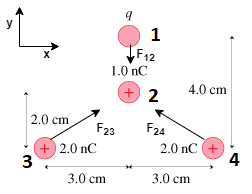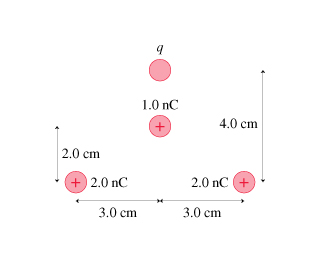# Problem: The net force on the 1.0 nC in the figure charge is zero.  What is q?

###### FREE Expert Solution

Coulomb's force,

$\overline{){\mathbf{F}}{\mathbf{=}}\frac{\mathbf{k}{\mathbf{q}}_{\mathbf{1}}{\mathbf{q}}_{\mathbf{2}}}{{\mathbf{r}}^{\mathbf{2}}}}$

Vector magnitude,

$\overline{)\mathbf{|}\stackrel{\mathbf{⇀}}{\mathbf{r}}\mathbf{|}{\mathbf{=}}\sqrt{{{\mathbf{r}}_{\mathbf{x}}}^{\mathbf{2}}\mathbf{+}{{\mathbf{r}}_{\mathbf{y}}}^{\mathbf{2}}}}$

Components of  a vector,

Direction (measured from x-axis),q should repel the 1.0 nC charge. Force F12 is along the y-axis in the negative direction.

The sum of the y-components of the force due to the two 2.0 nC charges should have the same magnitude as the force due to q.

The magnitude of r is the same for the two 2.0 nC charges,

r = 3.0 cm i + 2.0 cm j = 0.03 m i + 0.02 m j

$\mathbit{r}\mathbf{=}\sqrt{\mathbf{0}\mathbf{.}{\mathbf{03}}^{\mathbf{2}}\mathbf{+}\mathbf{0}\mathbf{.}{\mathbf{02}}^{\mathbf{2}}}$

r = 0.0361 m

100% (42 ratings)###### Problem Details

The net force on the 1.0 nC in the figure charge is zero.  What is q?